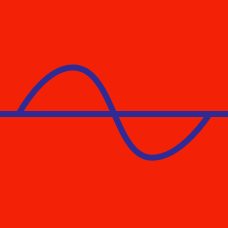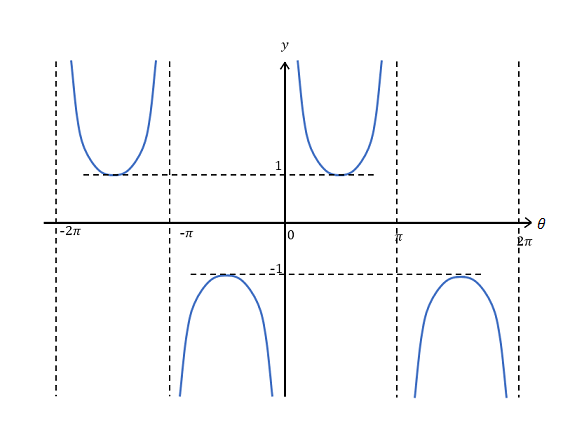Geometry

# Cosec and Sec Graphs

What is the minimum value of the function $y= \sec\theta+13$ in the interval $-\frac{\pi}{2} < \theta < \frac{\pi}{2} ?$Which of the following functions corresponds to the above graph?

For what value(s) of $\theta$ do we have $\sin\theta = \csc\theta$ and $0 \leq \theta < 2\pi ?$

What is the periodicity of the function $y=\sec\theta ?$

What is the sum of the $x$-coordinates of the intersection points of the two graphs $y=\csc x$ and $y= x ,$ in the interval $-\pi < x < \pi ?$

×